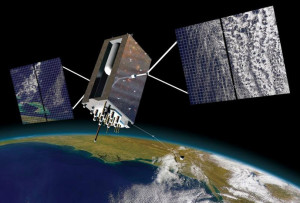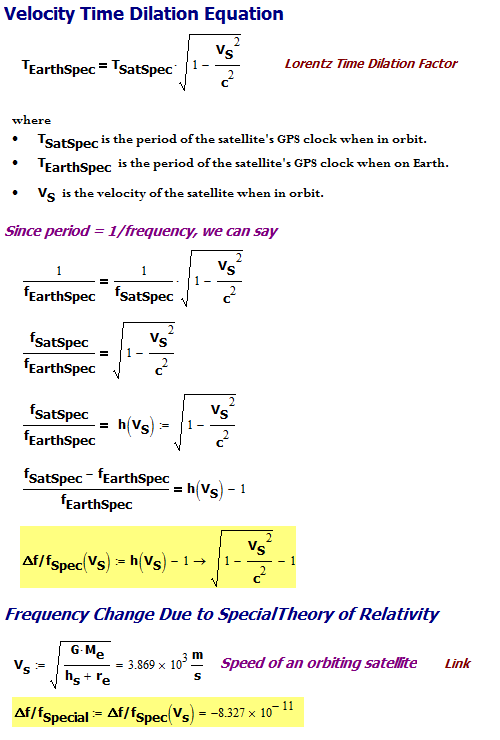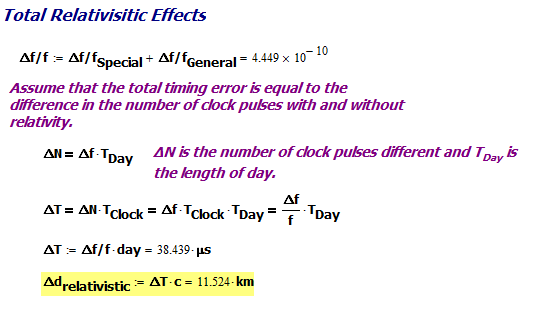# Compensating GPS Clocks for the Effects of Relativity

What you are will show in what you do.

— Edison

## IntroductionFigure 1: Block 3 GPS Satellite. These satellites
have changed many aspects of our lives,
everything from land surveying to car driving
and flying airplanes (Source).

I occasionally read articles on Stumbleupon and I came across an interesting article called "8 shocking things we learned from Stephen Hawking's book" – the book they are referring to is called The Grand Design. I personally would not call the statements in the article shocking, but that is just my opinion.

However, there were two factoids that I thought were interesting and I would write posts about. This post will be about the following statement on relativity and the GPS system (Figure 1).

If general relativity were not taken into account in GPS satellite navigation systems, errors in global positions would accumulate at a rate of about ten kilometers each day.

I read a paper quite a while ago on the topic and I recalled that there is ~14 kilometer per day error due to general relativity effects, and ~2 km per day of error in the opposite direction for special relativity, for a total error of ~12 km per day. So I thought I would work through the math behind these effects and present the results here.

## Background

### General Information

If you are looking for more background on GPS errors, the Wikipedia as a very good article on the magnitude of the relativistic errors in GPS on this page. My approach here will be to work through the equations and material shown in Figure 2 of this post, which presents a slightly different viewpoint on the same material. Figure 2 contains an equation for computing the frequency shift due to general relativity that I have not worked with before and I will derive it as part of my work here.

### Infographic on GPS Satellites

Figure 2 shows an infographic from Hugo Fruehauf, one of the key GPS developers. In this graphic, he mentions a daily timing correction of 38.6 µsec for relativistic effects. When I heard this timing correction, I quickly multiplied it by the speed of light to get the equivalent distance error (Equation 1).

 Eq. 1 $latex \displaystyle {{d}_{r}}=c\cdot \Delta {{t}_{r}}=3.8\cdot {{10}^{8}}{\frac{\text{m}}{\text{s}}}\cdot 38.6\mu\text{sec = 11}\text{.6 km}&s=2$

where

• Δtr is the daily GPS timing correction required for the combined effects of general and special relativity.
• dr is the distance error due to the combined relativistic effects.
• c is the speed of light in a vacuum.

### General Relativity Effect on GPS Clock Frequency

Because a clock on earth is subject to a stronger gravitational field than the satellite, the clock on the satellite will appear outrun the same clock on the earth. We can use Equation 2 to determine the impact of gravitational time dilation on the clock frequency at different distances from the center of a gravitational field.

 Eq. 2 $latex \displaystyle {{T}_{{Gravity}}}={{T}_{{NoGravity}}}\sqrt{{1-\frac{{2 \cdot G \cdot M_e}}{{r \cdot {{c}^{2}}}}}}&s=2$

where

• TNoGravity is the clock's period infinitely far from any gravitational field.
• TGravity is the clock's period at distance r from the center of the gravitational field.
• c is the speed of light in a vacuum.
• Me is the mass of the Earth.
• G is the universal gravitational constant.

While the GPS clock is in orbit, we will need to know its period as measured from the Earth. However, the the influence of gravity will cause the apparent clock period to change when it moves from the Earth into orbit. We can compute the clock period shift due to the effects of general relativity by using Equation 2 and working with the differences in the clock periods at the radii of the Earth's surface and the satellite's orbit.

### Special Relativity Effect on GPS Clock Frequency

Because a clock on the satellite is moving relative to the same clock on the earth, the clock on the satellite appears to run slower than the same clock on earth. The clock period on the orbiting satellite (TSatSpec), is longer than the clock period (TEarthSpec) for the same clock at rest on Earth (Equation 3).

 Eq. 3 $latex \displaystyle {{T}_{{EarthSpec}}}={{T}_{{SatSpec}}}\cdot \sqrt{{1-\frac{{{{v}^{2}}}}{{{{c}^{2}}}}}}&s=2$

where

• v is the speed of the moving observer.
• TEarthSpec  is the clock period measured for a GPS clock on Earth.
• TSatSpec is the clock period for a GPS clock mounted on a "fast" satellite and measured by an observer on Earth.

The clock period correction required for special relativity is in the opposite direction as the correction for general relativity.

## Analysis

### Percentage Frequency Change Due to General Relativity

Figure 3 shows my derivation of a formula for the percentage frequency shift due to the effects of general relativity. My results agree with those shown in Figure 2.

### Percentage Frequency Change Due to Special Relativity

Figure 4 shows my derivation of the formulas and results for the percentage clock  frequency change caused by the effects of special relativity. My results agree with those shown in Figure 2.Figure 4: Derivation of Percentage Frequency Change Due to Special Relativity.

### Combined Frequency Change

Figure 5 shows the combined frequency error effects of general and special relativity and how they result in a distance error of about ~12 km per day.Figure 5: Combined Relativistic Effect on Clock Rate and Effective Distance Error.

## Conclusion

I was able to show that the combined effects of general and special relativity produce ~12 km worth of distance error per day into the GPS system that must be accounted for. This agrees with the quote from the Stumbleupon article.

For a very good history of the GPS program, see this document.

Save

### 5 Responses to Compensating GPS Clocks for the Effects of Relativity

1.Khushi says:

•mathscinotes says:

The relativity equations and orbital data are readily available from many locations – I use the Wikipedia. The basic GPS information is from this document.

mark

2.Cliff Gold says:

Isn't the satellite in freefall, so does not experience any time dilation?

•big smart says:

have you seen any satellite hit the ground recently?

•mathscinotes says:

There are two forms of time dilation: gravitational (clocks go slower nearer a massive object) and Lorentz time dilation (clocks go slower due to their relative motion). Assuming you are a distant and stationary observer, an object falling toward a mass will experience both gravitational and Lorentz time dilation relative to you.

There is a good Quora response on this topic.